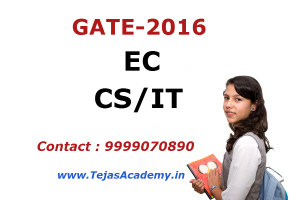# Gate coaching in Delhi schedule 28 July 2018Gate coaching in Delhi schedule 28 July 2018

Tejas Engineers Academy is providing you Gate coaching in Delhi for ECE , EEE, CSE and IT. Following is the schedule of Gate coaching batch on Tejas Engineers Academy

Time :-4:30 PM to 7:00 PM

Topics:- Linear Algebra, Numerical Methods.

Venue:- Tejas Engineers Academy Meenakshi Garden.

Previous lecture of Gate coaching was based on Engineering Mathematics.

If you are a final year or a pass out student of ECE/CSE/IT and looking for a good institute for Gate coaching in Delhi then you can come on above schedule to attend demo class. In class we will also provide you detailed brochure and class notes.

Explanation:- In this lecture we will cover Linear algebra consisting of Determinants and Matrices. We will start with the concept of rank of a matrix. Rank of a matrix can be found by converting the matrix in normal form. Whenever a matrix is expressed in normal form then its rank is the order of minimum square matrix. You can expect one question of finding rank of a matrix in coming Gate exam.

Then we will cover the details of finding consistency information of systems of linear equations. In this topic a system of equations is given in which we have to judge whether the given equation is consistent, inconsistent or trivial. This can be done by finding the rank of coefficient matrix of determinant.

We will then find characteristic equation of matrix and eigen values of a matrix. These are very important when we have to find eigen vectors of matrix. The study of eigen vectors is very important and you can expect one question in coming Gate exam.

Then we will cover Caley Hamilton theorem. This theorem is very useful for finding the inverse of matrix.